Next: Introduction to SuperDARN Up: The SuperDARN HF radar Previous: The SuperDARN HF radar

# Coherent Scatter Radar and the Scatter Theorem

Several types of scatter radar techniques are used for upper atmosphere research. Coherent scatter radar is a volume scattering technique whereby the radar detects energy scattered from within a medium when there are regular spatial variations of the refractive index due to irregularities. This is the analogue of Bragg scattering of X-rays from crystals. The ionosphere contains irregularities of many different sizes and in general a small fraction of the incident energy is scattered at each boundary. Signals backscattered from plasma wave irregularities with a spacing of half the radar wavelength will reinforce by constructive interference in the direction back to the radar and can produce a signal strong enough to be detected by the receiver. Thus, a radar of wavelength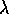will effectively select from the scattering medium the spatial component of wavelengthalong the radar beam (a more detailed derivation of this property follows). The term ``coherent'' applies to the constructive interference possible when there is a scattering structure with an organized spatial content at half the radar wavelength.

The scatter theorem describes the signals that can be measured by a coherent scatter radar. Figure 3.1 (left) shows the geometry used in the derivation of the scatter theorem for a bistatic radar system.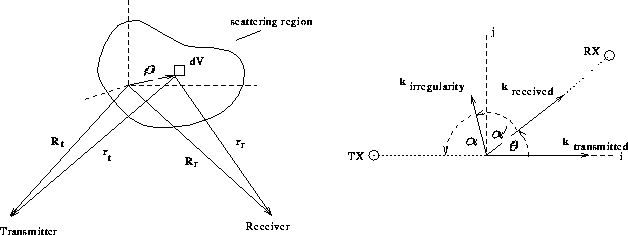Figure 3.1: Geometry of scatter theorem analysis

The origin of the coordinate system lies within the scattering region under observation,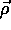points to a position within this region,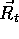and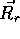are the positions of the transmitter and the receiver, respectively. They are fixed in space and can be considered constant in this derivation. We introduce further the vectors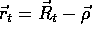and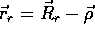. Considered is a volume element dV at the position. The electric field produced by the transmitter is given by:where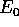is the electric field at the transmitter,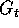is the transmitter antenna voltage gain and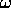is the angular frequency of the wave.The electric field at the receiverfrom the volume element dV is then given by: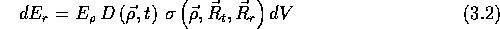where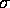is the amplitude scattering cross section of electrons for signals incident along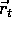and scattered along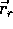and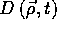is the electron density atand time t. To simplify this equation it is assumed that for a narrow beam, the voltage gain function for the transmitting and receiving antennas can be set to constantsand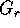respectively. A similar simplification is done with the scattering cross sectionwhich is set to a constant, since all spatial information is contained in the electron density term D. The differential voltage produced at the receiver input by volume element dV of the scatterer is then: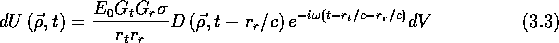Assuming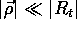and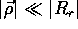, performing a Taylor expansion onand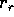and neglecting quadratic terms, one obtains the following approximations for the transmitter (t) and receiver (r) distances: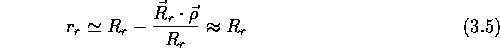This approximation can now be used to rewrite the amplitude term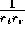, but not the phase term. Using the unit vectors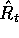and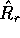, equation 3.3 becomes: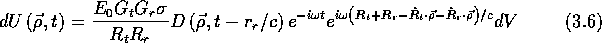We express the electron densitythrough its Fourier component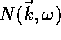. Thus if the transform is denoted by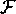the definition of N is: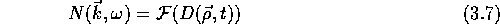That is, by the inverse transform,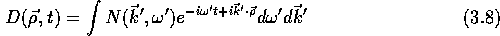or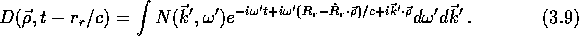Substituting Equation 3.9 into Equation 3.6 yields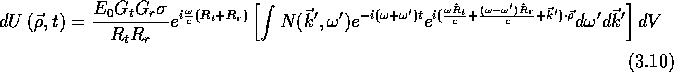In these steps we also used the fact that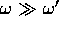. If we integrate over the volume where backscatter occurs, the-dependent exponential term can be approximated by a delta function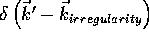, where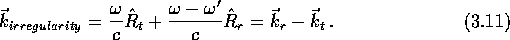This means we select the component of N that corresponds to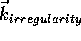. After the integration over, taking the delta function into account, the final result can be written as followswhere C denotes some constant.

This shows that the radar is sensitive to the Fourier components of the electron density fluctuation with wave vector, corresponding to the wavelength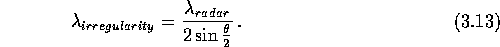whereis the angle betweenand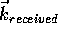. This important property can be derived by considering the following equation derived from the conservation of momentum and energy in two dimensions (see Figure 3.1, right):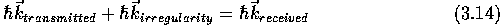and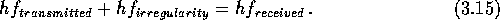Rewriting Equation 3.14 in terms of the wavelengthfor the x and y components gives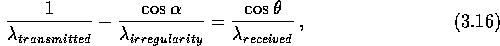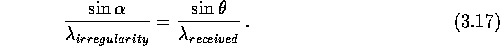Similary Equation 3.15 becomes (using 3.17)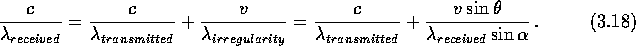Hence using Equation 3.16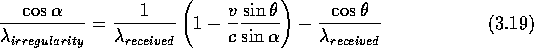and Equation 3.17 we get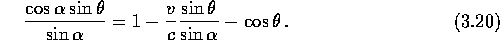Using some trigonometric identities this can be written as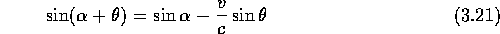and since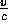is small, it follows thatBut since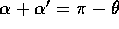we arrive at the property that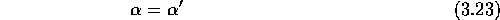which means that the angle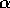whichmakes with the negative x-axis is along the bisector of the transmitter and receiver directions. Using this fact we can write Equation 3.14 as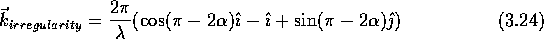whereis the unit vector pointing along the incident direction and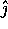is perpendicular to. This can be simplified using the following trigonometric identities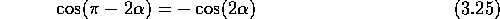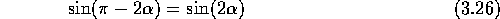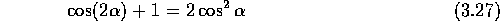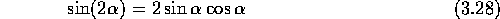to yield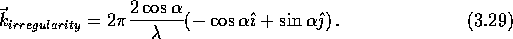By comparing the left and right side of this equation it follows that the direction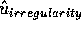and wavelength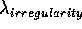is described by: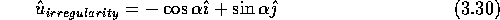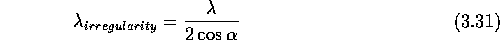We can convertto the anglethat was used earlier using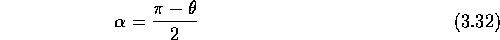which yields Equation 3.13 again. For a monostatic backscatter radar, with transmitting and receiving antennas at the same location,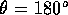and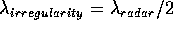.Next: Introduction to SuperDARN Up: The SuperDARN HF radar Previous: The SuperDARN HF radar

Andreas Schiffler
Wed Oct 9 10:05:17 CST 1996